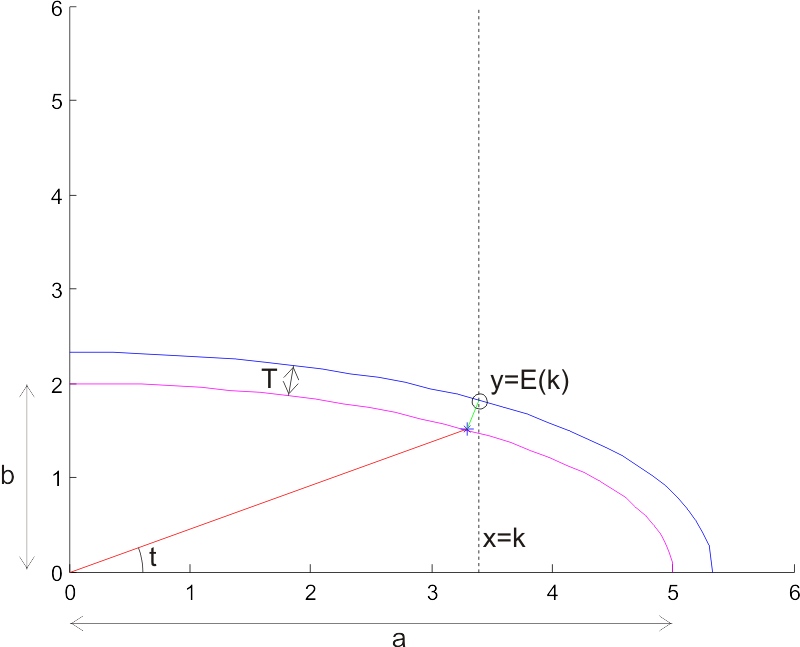# Offset Ellipse

Continuing with some random thoughts on CAM algorithms, here's a diagram I drew in response to a question on offset ellipses posed by Julian Todd.(click image for high-resolution version)
The task is to find an offset ellipse to a given ellipse (magenta). The offset ellipse (blue) should lie a distance T, measured along the normal to the ellipse (I've drawn one normal of length T in green), from the original ellipse. Drawing the offset ellipse is simple, but it's a bit harder to find a point on the offset for a given x-coordinate x=k. I've marked this point with a circle, and its coordinates are (k, E(k)), so E(k) is a function that returns the sought y-coordinate.

I took Julian's advice of using a numerical method with respect to the geometry (not neccessarily cartesian coordinates), and parametrized the ellipse as a function of an angle t. So points along the ellipse lie at:

ex=a*cos(t)
ey=b*sin(t)

Where a and b are the major and minor axes of the ellipse, and t is an angle between 0 and 2pi. At every point there's a normal vector

nx = b*cos(t)
ny = a*sin(t)

To find points on the sought offset ellipse, we need to scale this normal so it's length is T. Each normal has a length

l=sqrt(nx^2 + ny^2)

So the sought normal vector is

nx_T = (nx/l)*T
ny_T = (ny/l)*T

or more explicitly

nx_T = ( b*cos(t) / sqrt((b*cos(t) )^2 + (a*sin(t))^2) ) * T
ny_T = ( a*sin(t) / sqrt((b*cos(t) )^2 + (a*sin(t))^2) ) * T

Now, points on the offset ellipse lie at

oe_x = ex + nx_T
oe_y = ey + ny_T

and we need to find the particular t angle which results in a point (oe_x, oe_y) for which oe_x = k holds. Inserting the above expressions, this happens when:

(a+T*b/sqrt( ( b*cost(t) )^2 + ( a*sin(t) )^2 ))*cos(t) -k = 0

I haven't looked for an analytic solution to this equation, but plotting it for a few test cases seems to indicate that it's fairly 'benign', and Matlab's fzero function finds a solution very quickly. If we call the solution to this equation tk, the sought point on the offset ellipse is

E(k) = oe_y(tk)

Here are two Matlab scripts I used to plot this figure: ellipsetest.m is the main program, and oe.m is used when solving the equation.

## 6 thoughts on “Offset Ellipse”

1.Julian Todd says:

I'll be publishing a C++ version of my solution in the next few weeks once I get everything else to do with the cutter location working.

The main thing is to avoid using sin and cos, which are slow functions, because you can do everything using nothing more complex than the sqrt.

2.Proctologically Violated says:

To Julian:

The reason trig functions are slow is because calculator/processors use very long power series to generate them. You can use much shorter power series to get .0001 resolution, and the calc time should be proportionately faster--if speed is even an issue at all, these days.
Spreadsheets generate 15 decimal places for sin/cos, iirc, and they seem pretty fast to me!

To the main article:

Can't you generate "concentric ellipses" by simply adjusting a, b, and c in x2/a2 + y2/b2 = c??
--
Mr. PV

3.Administrator says:

Mr PV, I think the idea is that you want the point that is a distance d along the normal from the original ellipse. your concentric ellipse certainly contains that point, but it doesn't help to identify the particular point that is along the normal from the original point.

4.Rudi says:

But the question remains whether the offset of an ellipse is an ellipse? The solution you present gives a point normal to the given ellipse at a given distance, but if these points were to lie on an ellipse, it can only be an ellipse with axes a' = a + T and b' = b + T, such that
oe_x=(a+T)*cos(t')
oe_y=(b+T)*sin(t')
This can easily be checked for t = 0, PI/2, ... It's a bit harder to check it for any angle t (analytically thus). I did try it for a random t value, and it didn't seem the case, though I may have made some mistake.

5.Administrator says:

I believe the only types of curves that are simple to offset, since their offset is the same type as the curve, are line segments and arc segments.

anything more complex (like an ellipse, a bezier curve etc.) will have an offset that cannot generally be described with the same kind of equation as the original curve.

6.joshua dachs says:

An offset ellipse (also known as an Elliptic Parallel) is absolutely NOT an ellipse.